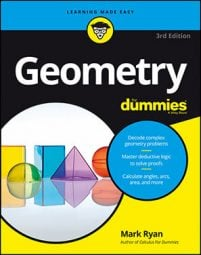##### Geometry For DummiesWhen writing a two-column proof, you need to spell out every little step as if you had to make the logic clear to a computer. To do this, you can use if-then logic to move from the givens to the final conclusion.

The following example starts with 'given' information, and shows the first few lines of the proof.

And here's the proof diagram.

Now check out the first few lines in the two-column proof.

Notice how the if-then sentence structure of each reason in the proof shows you how each statement "knocks over" another statement like a domino. Consider the reason "if two segments are perpendicular, then they form right angles." The perpendicular domino (statement 1) knocks over the right-angle domino (statement 2).

Add the third line of the proof.

Reason 3 explains how the right-angle domino (statement 2) knocks over the congruent angle domino (statement 3). This process continues throughout the whole proof, but it's not always as simple as 1 knocks over 2, 2 knocks over 3, 3 knocks over 4, and so on. Sometimes you need two statements to knock over another, and sometimes you skip statements; in another proof, for example, statement 3 might knock over statement 5. Focusing on the if-then logic of a proof helps you see how the whole proof fits together.

Make sure that the if-then structure of your reasons is correct:

• The idea or ideas in the if clause of a reason must appear in the statement column somewhere above the line of that reason.
• The single idea in the then clause of a reason must be the same idea that's in the statement directly across from the reason.
Look back at the first two lines of the proof. Because statement 1 is the only statement above reason 2, it's the only place you can look for the ideas that go in the if clause of reason 2. So if you begin this proof by putting the two pairs of perpendicular segments in statement 1, then you have to use that information in reason 2, which must therefore begin "if segments are perpendicular, then . . ."

Now say you didn't know what to put in statement 2. The if-then structure of reason 2 helps you out. Because reason 2 begins "if two segments are perpendicular . . ." you'd ask yourself, "Well, what happens when two segments are perpendicular?" The answer, of course, is that right angles are formed. The right-angle idea must therefore go in the then clause of reason 2 and right across from it in statement 2.

Okay, now what? Well, think about reason 3. One way it could begin is with the right angles from statement 2. The if clause of reason 3 might be "if two angles are right angles …" Can you finish that? Of course: If two angles are right angles, then they're congruent. So that's it: You've got reason 3, and statement 3 must contain the idea from the then clause of reason 3, the congruence of right angles.